# 说明

php的数组可以说很强大，千万不要把Go的数组看做php，php数组应该是包含了Go的数组和切片。

# 声明和初始化

## 数组

Go 语言数组声明需要指定元素类型及元素个数，也就是说，在 Go 语言中，数组是固定长度的、同一类型的数据集合

``````var variable_name [SIZE] variable_type
//多维数组
var variable_name [SIZE1][SIZE2]...[SIZEN] variable_type
``````

``````a := int{1,2,3,4,5}//这种情况下，Go 会在编译期自动计算出数组长度（3）

var threedim int //三维数组
``````

``````a := int{1: 3, 3: 7}
//这样表示数组长度为5，下标为1的值为3，下标为3的值为7，其他用0填充
``````

## 切片

len() 和 cap() 返回结果可相同和不同。切片的定义：

``````var identifier []type
``````

``````var slice1 []type = make([]type, len)

slice1 := make([]type, len)
``````
``````package main

import (
"fmt"
)

func main() {
//nil切片
var nilSlice [] int
//空切片
emptySlice := [] int {}
//声明切片，打印结果
var slice = make([]int, 3, 5)
// 初始化一个长度为5的切片
slice1 := make([]int, 5)
// 初始化一个长度为5的切片，容量为10
capacitySlice := make([]int, 5, 10)
//数组截取成切片
var arr = int{1, 2, 3, 5, 4}
// 基于数组创建数组切片,这个切片，从下标为3开始，到下标为6，截取数组
arrSlice := arr[3:6]
//获取整个数组作为切片
all := arr[:]

fmt.Printf("nilSlice：len=%d cap=%d nil切片slice=%v\n", len(nilSlice), cap(nilSlice), nilSlice)
fmt.Printf("emptySlice：len=%d cap=%d 空切片slice=%v\n", len(emptySlice), cap(emptySlice), emptySlice)
fmt.Printf("slice：len=%d cap=%d 切片slice=%v\n", len(slice), cap(slice), slice)
fmt.Printf("slice1：len=%d cap=%d 切片slice=%v\n", len(slice1), cap(slice1), slice1)
fmt.Printf("capacitySlice：len=%d cap=%d 切片slice=%v\n", len(capacitySlice), cap(capacitySlice), capacitySlice)
fmt.Printf("arrSlice：len=%d cap=%d 数组切片slice=%v\n", len(arrSlice), cap(arrSlice), arrSlice)
fmt.Printf("all：len=%d cap=%d 整个数组切片slice=%v\n", len(all), cap(all), all)
}
``````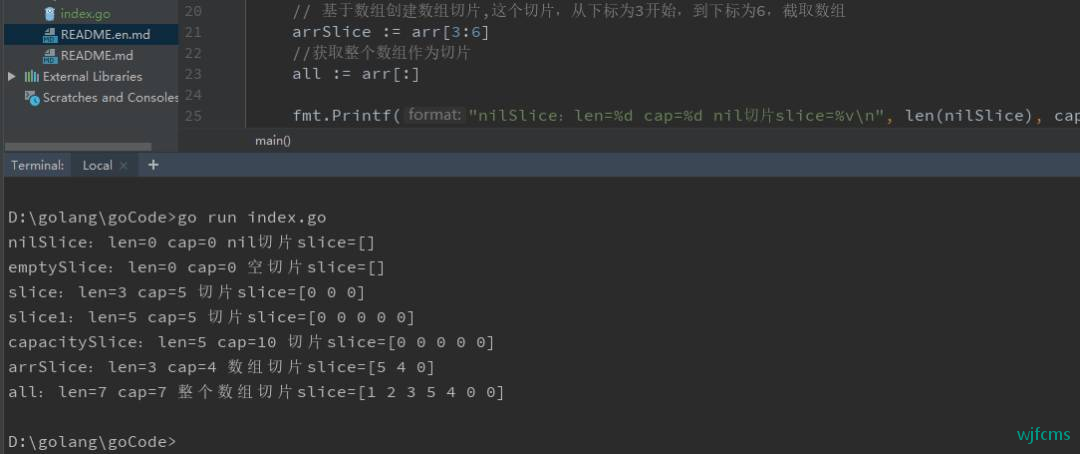nilSlice：len=0 cap=0 nil切片slice=[]
emptySlice：len=0 cap=0 空切片slice=[]
slice：len=3 cap=5 切片slice=[0 0 0]
slice1：len=5 cap=5 切片slice=[0 0 0 0 0]
capacitySlice：len=5 cap=10 切片slice=[0 0 0 0 0]
arrSlice：len=3 cap=4 数组切片slice=[5 4 0]
all：len=7 cap=7 整个数组切片slice=[1 2 3 5 4 0 0]

# 访问和遍历数组

## 数组和切片访问和遍历相同

Go访问数组(切片)元素和php一样，都是以下标去访问，并且都是从0开始，到len（arr-1）结束。

Go的for遍历和php的for循环类似，这里不做过多说明。

``````for i := 0; i < len(summer); i++ {
fmt.Println("summer[", i, "] =", summer[i])
}
``````

Go 语言还提供了一个关键字 range（有点类似php的foreach），用于快速遍历元素：

``````for i, v := range arr {
fmt.Println("下标：", i, "值：", v)
}

for _, v := range arr {
// 这样就只获取值，不获取下标
}

for i := range arr {
// 这样就只获取下标，不获取值
}
``````

# 操作

## 容量(函数cap())和实际长度(函数len())

``````var arr = float32{100.0, 2.0, 3.4, 7.0, 50.0}
//初始化一个切片{0,0,0,0,},其容量为10
slice := make([]int, 5, 10)
fmt.Printf("arr：len=%d cap=%d arr=%v\n", len(arr), cap(arr), arr)
fmt.Printf("slice：len=%d cap=%d slice=%v\n", len(slice), cap(slice), slice)
``````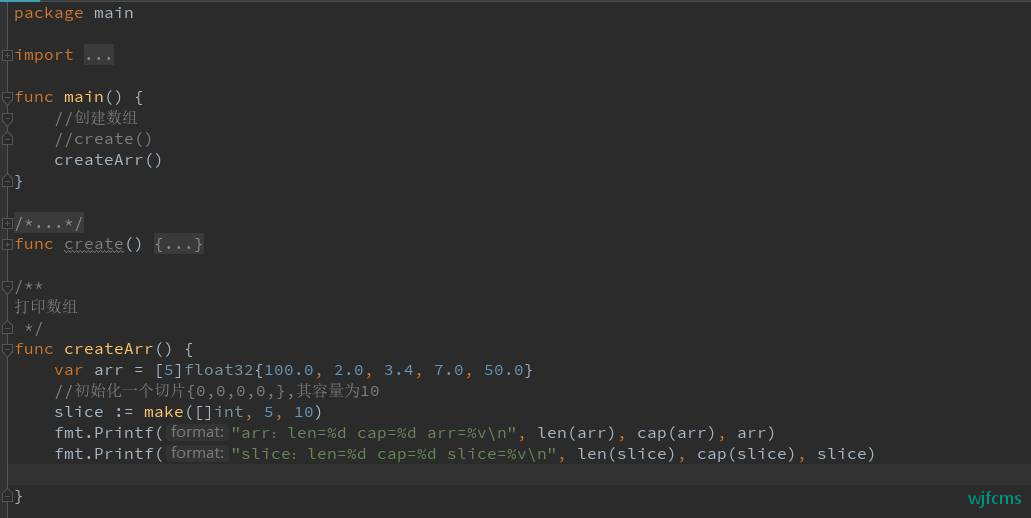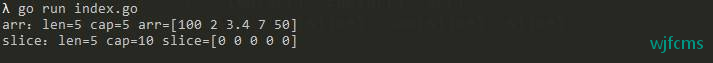λ go run index.go
arr：len=5 cap=5 arr=[100 2 3.4 7 50]
slice：len=5 cap=10 slice=[0 0 0 0 0]

## 数组

``````arr = 100
``````

## 切片

### 追加与复制

``````var numbers []int
printSlice(numbers)
/* 允许追加空切片 */
numbers = append(numbers, 0)
printSlice(numbers)
/* 同时添加多个元素 */
numbers = append(numbers, 2,3,4)
printSlice(numbers)
/* 创建切片 numbers1 是之前切片的两倍容量 如果加入的两个数组切片不一样大，就会按其中较小的那个数组切片的元素个数进行复制。*/
numbers1 := make([]int, len(numbers), (cap(numbers))*2)
/* 拷贝 numbers 的内容到 numbers1 */
copy(numbers1,numbers)
printSlice(numbers1)
``````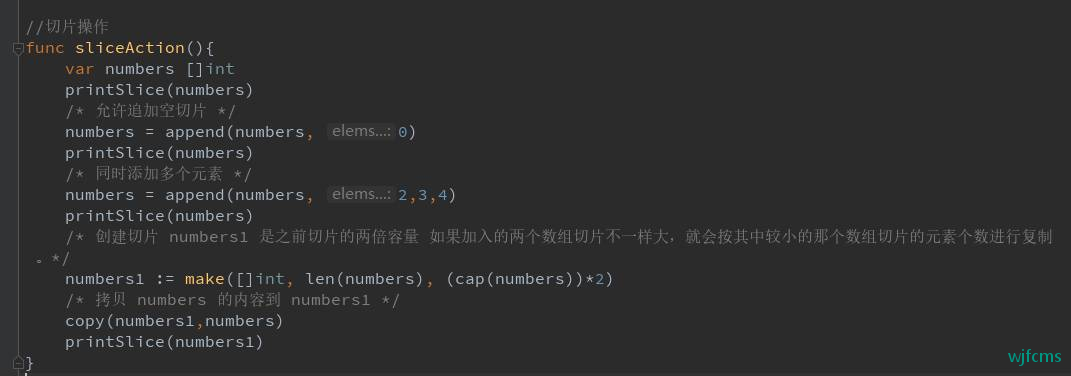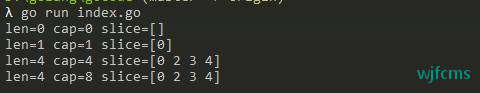λ go run index.go
len=0 cap=0 slice=[]
len=1 cap=1 slice=
len=4 cap=4 slice=[0 2 3 4]
len=4 cap=8 slice=[0 2 3 4]

### 截取

``````/* 创建切片 */
numbers := []int{0,1,2,3,4,5,6,7,8}
printSlice(numbers)
/* 打印原始切片 */
fmt.Println("numbers ==", numbers)
/* 打印子切片从索引1(包含) 到索引4(不包含)*/
fmt.Println("numbers[1:4] ==", numbers[1:4])
/* 默认下限为 0*/
fmt.Println("numbers[:3] ==", numbers[:3])
/* 默认上限为 len(s)*/
fmt.Println("numbers[4:] ==", numbers[4:])
numbers1 := make([]int,0,5)
printSlice(numbers1)
/* 打印子切片从索引  0(包含) 到索引 2(不包含) */
number2 := numbers[:2]
printSlice(number2)
/* 打印子切片从索引 2(包含) 到索引 5(不包含) */
number3 := numbers[2:5]
printSlice(number3)
``````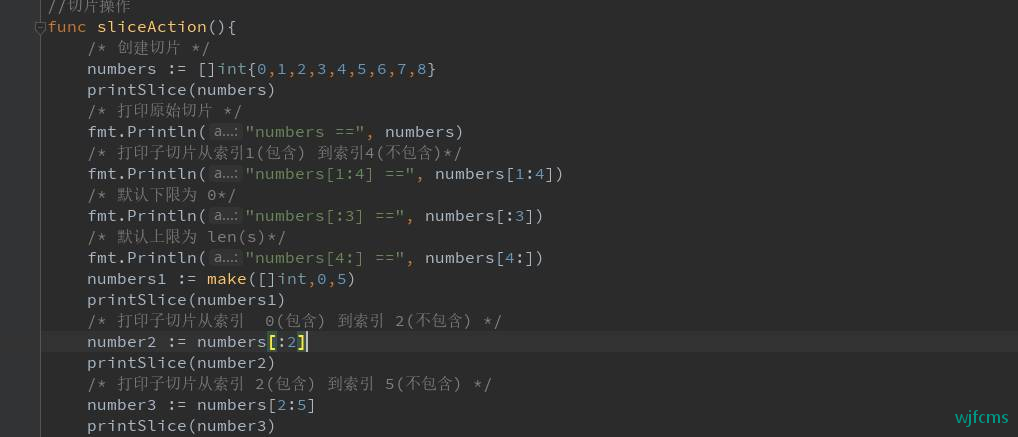λ go run index.go
len=9 cap=9 slice=[0 1 2 3 4 5 6 7 8]
numbers == [0 1 2 3 4 5 6 7 8]
numbers[1:4] == [1 2 3]
numbers[:3] == [0 1 2]
numbers[4:] == [4 5 6 7 8]
len=0 cap=5 slice=[]
len=2 cap=9 slice=[0 1]
len=3 cap=7 slice=[2 3 4]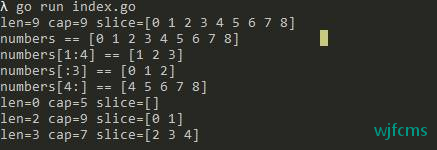{{ comment.content }}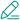@ {{ child.answered_user_name }}:{{ child.content }}• 微信• 如果你觉得本站很棒，可以通过扫码支付打赏哦！

•微信收款码
•支付宝收款码